# PSAT Math : Geometry

## Example Questions

1 2 59 60 61 62 63 64 65 67 Next →

### Example Question #21 : Rectangles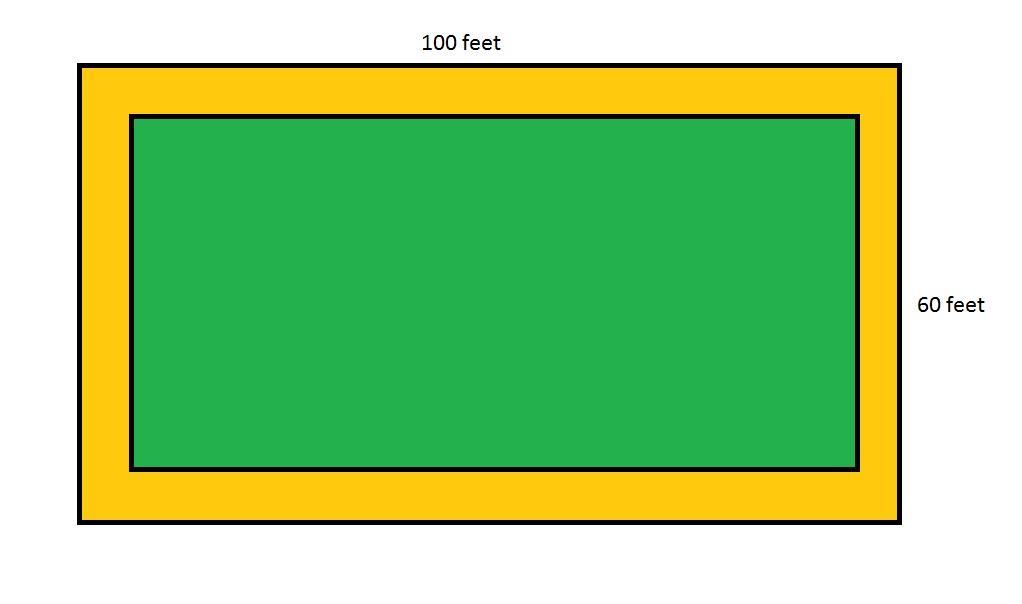Note:  Figure NOT drawn to scale

Refer to the above figure, which shows a rectangular garden (in green) surrounded by a dirt path (in orange) eight feet wide throughout. What is the area of that dirt path?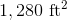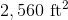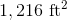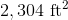The correct area is not given among the other responses.Explanation:

The dirt path can be seen as the region between two rectangles. The outer rectangle has length and width 100 feet and 60 feet, respectively, so its area is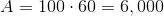square feet.

The inner rectangle has length and width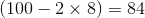feet and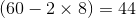feet, respectively, so its area is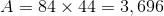square feet.

The area of the path is the difference of the two: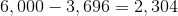square feet.

### Example Question #664 : Geometry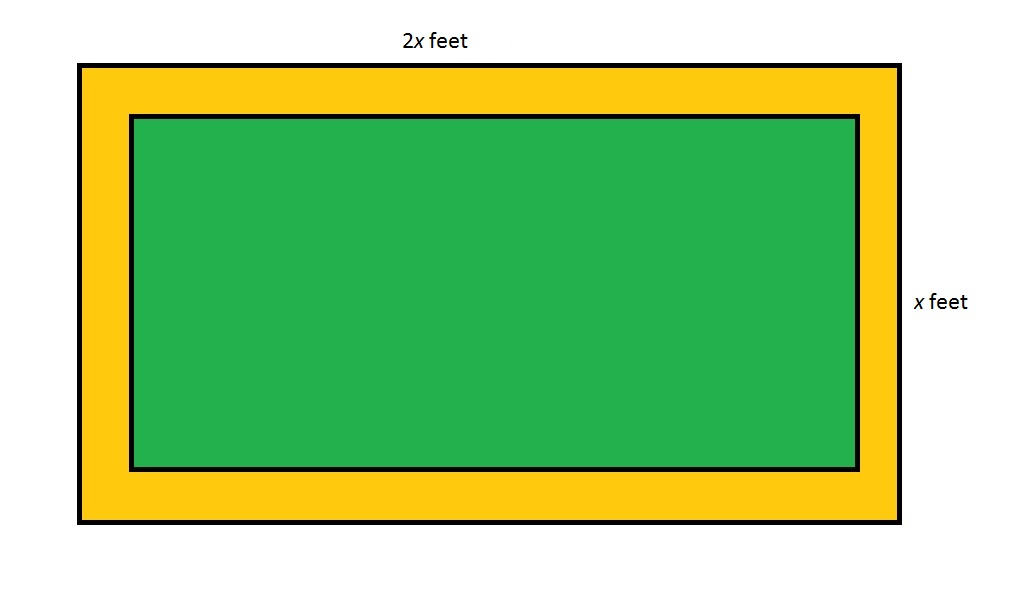Refer to the above figure, which shows a rectangular garden (in green) surrounded by a dirt path (in orange). The dirt path is seven feet wide throughout. Which of the following polynomials gives the area of the dirt path in square feet?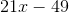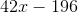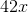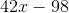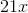Explanation:

The area of the dirt path is the difference between the areas of the outer and inner rectangles.

The outer rectangle has area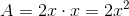The area of the inner rectangle can be found as follows:

The length of the garden is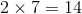feet less than that of the entire lot, or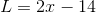;

The width of the garden isless than that of the entire lot, or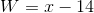;

The area of the garden is their product: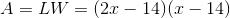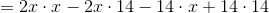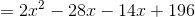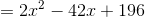Now, subtract the areas: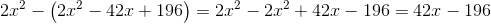### Example Question #22 : How To Find The Area Of A Rectangle

Two circles of a radius of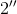each sit inside a square with a side length of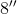.  If the circles do not overlap, what is the area outside of the circles, but within the square?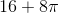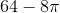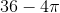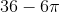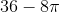Explanation:

The area of a square =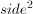The area of a circle is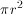Area  = Area of Square2(Area of Circle) =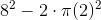### Example Question #23 : How To Find The Area Of A Rectangle

If the area Rectangle A islarger than Rectangle B and the sides of Rectangle A areand, what is the area of Rectangle B?Explanation: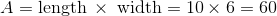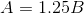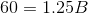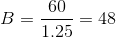### Example Question #2 : How To Find The Perimeter Of A Parallelogram

ABCD is a parallelogram. BD = 5. The angles of triangle ABD are all equal. What is the perimeter of the parallelogram?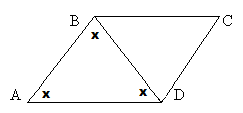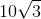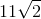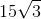Explanation:

If all of the angles in triangle ABD are equal and line BD divides the parallelogram, then all angles in triangle BDC must be equal as well.

We now have two equilateral triangles, so all sides of the triangles will be equal.

All sides therefore equal 5.

5+5+5+5 = 20

1 2 59 60 61 62 63 64 65 67 Next →

### All PSAT Math Resources